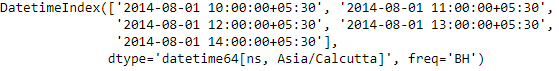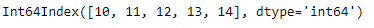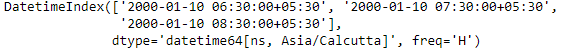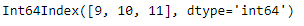Open in App
Not now

# Python | Pandas DatetimeIndex.hour

• Last Updated : 24 Dec, 2018

Python is a great language for doing data analysis, primarily because of the fantastic ecosystem of data-centric python packages. Pandas is one of those packages and makes importing and analyzing data much easier.

Pandas` DatetimeIndex.hour` attribute outputs an Index object containing the hour values present in each of the entries of the DatetimeIndex object.

Syntax: DatetimeIndex.hour

Return: Index containing hours.

Example #1: Use `DatetimeIndex.hour` attribute to find the hour values present in the DatetimeIndex object.

 `# importing pandas as pd``import` `pandas as pd`` ` `# Create the DatetimeIndex``# Here the 'BH' represents business hour frequency``didx ``=` `pd.DatetimeIndex(start ``=``'2014-08-01 10:00'``, freq ``=``'BH'``, ``                             ``periods ``=` `5``, tz ``=``'Asia/Calcutta'``)`` ` `# Print the DatetimeIndex``print``(didx)`

Output :Now we want to find all the hour values present in the DatetimeIndex object.

 `# find all the hour values present in the object``didx.hour`

Output :As we can see in the output, the function has returned an Index object containing the hour values present in each entry of the DatetimeIndex object.

Example #2: Use `DatetimeIndex.hour` attribute to find the hour values present in the DatetimeIndex object.

 `# importing pandas as pd``import` `pandas as pd`` ` `# Create the DatetimeIndex``# Here the 'H' represents hourly frequency``didx ``=` `pd.DatetimeIndex(start ``=``'2000-01-10 06:30'``, freq ``=``'H'``, ``                            ``periods ``=` `3``, tz ``=``'Asia/Calcutta'``)`` ` `# Print the DatetimeIndex``print``(didx)`

Output :Now we want to find all the hour values present in the DatetimeIndex object.

 `# find all the hour values present in the object``didx.hour`

Output :As we can see in the output, the function has returned an Index object containing the hour values present in each entry of the DatetimeIndex object.

My Personal Notes arrow_drop_up